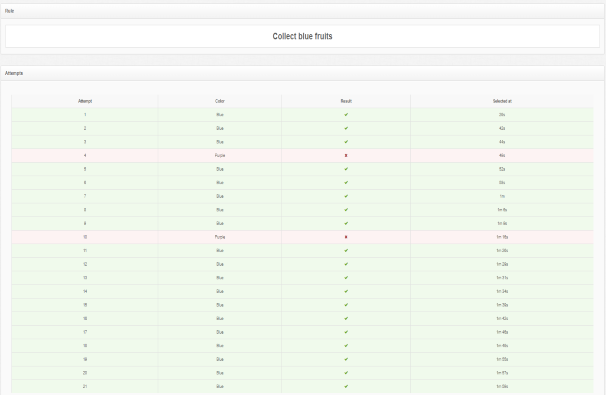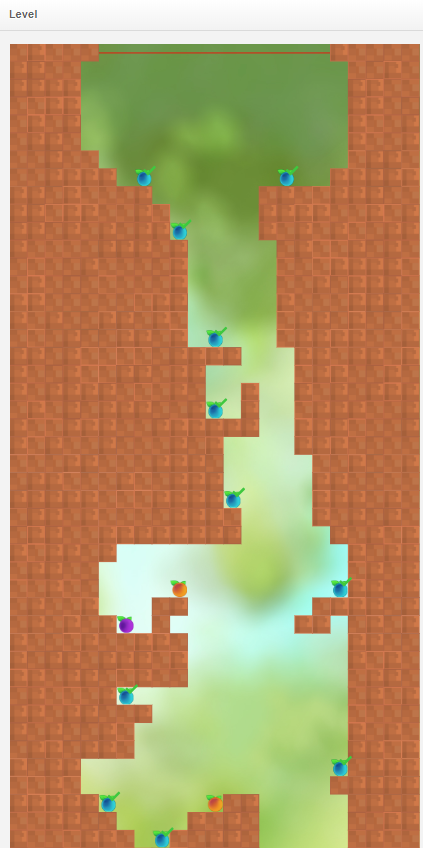### Woolly Strike

“Woolly Strike” encourages students to practice generating and filling in number patterns following a set of rules. Simultaneously, it helps students to improve their bilateral upper body coordination skills as they use both hands in a controlled manner with confidence and balance. Given a rule such as, add three, the student’s task is to collect the objects that are labeled with the correct corresponding numbers, thus generating a numerical pattern. Students develop competency in modeling relationships between numbers, and demonstrate their understanding of addition, subtraction, multiplication, and division.

The game-play requests the child to stretch both hands at almost 90 degrees, similar to holding a pole or broomstick. By coordinating both hands with an equal amount of force to balance the wooly ball, the student identifies the correct answer and rolls it towards the number to fulfill the specific pattern, catching the item with the right number or color. In this way, the game aids in increasing the shoulders’ range of motion and improves posture. The teacher or therapist can configure the type and difficulty of the numerical patterns, with rules involving addition, subtraction, multiplication, and division. They can also adjust the game elements such as the length of each game or the number of lives the student has to play.

Are you ready to juggle the wooly ball and collect the correct numbers and objects?

## Supported goals in Common CoreCaliforniaFloridaNevadaNew YorkNorth CarolinaPennsylvaniaSouth CarolinaTenesseeTexasVirginia

CCSS.MATH.CONTENT.4.OA.C.5
Math
Generate a number or shape pattern that follows a given rule. Identify apparent features of the pattern that were not explicit in the rule itself. For example, given the rule "Add 3" and the starting number 1, generate terms in the resulting sequence and observe that the terms appear to alternate between odd and even numbers. Explain informally why the numbers will continue to alternate in this way.
CCSS.MATH.CONTENT.5.OA.B.3
Math
Generate two numerical patterns using two given rules. Identify apparent relationships between corresponding terms. Form ordered pairs consisting of corresponding terms from the two patterns, and graph the ordered pairs on a coordinate plane. For example, given the rule "Add 3" and the starting number 0, and given the rule "Add 6" and the starting number 0, generate terms in the resulting sequences, and observe that the terms in one sequence are twice the corresponding terms in the other sequence. Explain informally why this is so.
CA.MATH.5.OA.3
Math
Analyze patterns and relationships. Generate two numerical patterns using two given rules. Identify apparent relationships between corresponding terms. Form ordered pairs consisting of corresponding terms from the two patterns, and graph the ordered pairs on a coordinate plane. For example, given the rule “Add 3” and the starting number 0, and given the rule “Add 6” and the starting number 0, generate terms in the resulting sequences, and observe that the terms in one sequence are twice the corresponding terms in the other sequence. Explain informally why this is so.
CA.MATH.4.OA.5
Math
Generate and analyze patterns. Generate a number or shape pattern that follows a given rule. Identify apparent features of the pattern that were not explicit in the rule itself. For example, given the rule “Add 3” and the starting number 1, generate terms in the resulting sequence and observe that the terms appear to alternate between odd and even numbers. Explain informally why the numbers will continue to alternate in this way.
MAFS.4.OA.3.AP.5b
Math
Extend a numerical pattern when the rule is provided
MAFS.5.OA.2.AP.3b
Math
Graph ordered pairs on a coordinate plane when given a table that follows patterns rules
NVAC.MATH.4.OA.C.5
Math
Generate and analyze patterns. Generate a number or shape pattern that follows a given rule. Identify apparent features of the pattern that were not explicit in the rule itself. For example, given the rule “Add 3” and the starting number 1, generate terms in the resulting sequence and observe that the terms appear to alternate between odd and even numbers. Explain informally why the numbers will continue to alternate in this way.
NVAC.MATH.5.OA.B.3
Math
Analyze patterns and relationships. Generate two numerical patterns using two given rules. Identify apparent relationships between corresponding terms. Form ordered pairs consisting of corresponding terms from the two patterns, and graph the ordered pairs on a coordinate plane. For example, given the rule “Add 3” and the starting number 0, and given the rule “Add 6” and the starting number 0, generate terms in the resulting sequences, and observe that the terms in one sequence are twice the corresponding terms in the other sequence. Explain informally why this is so.
NY.MATH.4.OA.5
Math
Generate a number or shape pattern that follows a given rule. Identify apparent features of the pattern that were not explicit in the rule itself. For example, given the rule “Add 3” and the starting number 1, generate terms in the resulting sequence and observe that the terms appear to alternate between odd and even numbers. Explain informally why the numbers will continue to alternate in this way.
NY.MATH.5.OA.3
Math
Generate two numerical patterns using two given rules. Identify apparent relationships between corresponding terms. Form ordered pairs consisting of corresponding terms from the two patterns, and graph the ordered pairs on a coordinate plane. For example, given the rule “Add 3” and the starting number 0, and given the rule “Add 6” and the starting number 0, generate terms in the resulting sequences, and observe that the terms in one sequence are twice the corresponding terms in the other sequence. Explain informally why this is so.
NC.MATH.5.OA.3
Math
Generate two numerical patterns using two given rules. Identify apparent relationships between corresponding terms. Form ordered pairs consisting of corresponding terms from the two patterns. Graph the ordered pairs on a coordinate plane.
NC.MATH.4.OA.5
Math
Generate and analyze a number or shape pattern that follows a given rule.
PA.CC.MATH.5.OA.B.3
Math
Generate two numerical patterns using two given rules. Identify apparent relationships between corresponding terms. Form ordered pairs consisting of corresponding terms from the two patterns, and graph the ordered pairs on a coordinate plane. For example, given the rule "Add 3" and the starting number 0, and given the rule "Add 6" and the starting number 0, generate terms in the resulting sequences, and observe that the terms in one sequence are twice the corresponding terms in the other sequence. Explain informally why this is so.
PA.CC.MATH.4.OA.C.5
Math
Generate a number or shape pattern that follows a given rule. Identify apparent features of the pattern that were not explicit in the rule itself. For example, given the rule "Add 3" and the starting number 1, generate terms in the resulting sequence and observe that the terms appear to alternate between odd and even numbers. Explain informally why the numbers will continue to alternate in this way.
SC.MATH.5.ATO.3
Math
Investigate the relationship between two numerical patterns. Generate two numerical patterns given two rules and organize in tables. Translate the two numerical patterns into two sets of ordered pairs. Graph the two sets of ordered pairs on the same coordinate plane. Identify the relationship between the two numerical patterns.
SC.MATH.4.ATO.5
Math
Generate a number or shape pattern that follows a given rule and determine a term that appears later in the sequence.
No supported goals.
TEKS.MATH.5.4.C
Math
Generate a numerical pattern when given a rule in the form y=ax or y=x + a and graph;
VASOL.MATH.2.PFA.20
Math
The student will identify, create, and extend a wide variety of patterns.
VASOL.MATH.1.PFA.17
Math
The student will recognize, describe, extend, and create a wide variety of growing and repeating patterns.
VASOL.MATH.K.PFA.15
Math
The student will sort and classify objects according to attributes.
VASOL.MATH.K.PFA.16
Math
The student will identify, describe, and extend repeating patterns.
VASOL.MATH.4.PFA.15
Math
The student will recognize, create, and extend numerical and geometric patterns.
VASOL.MATH.5.PFA.17
Math
The student will describe the relationship found in a number pattern and express the relationship.

## Settings

### I CAN statement

Music

Disable/enable background music.

• On
• Off
Sound effects

Disable/enable sound effects while selecting an item.

• On
• Off

I CAN understand that there are sounds when I choose an item.

Select hand

Time to remain stable to select an item.

• Left
• Right

I CAN select the “Play” button with my left hand.  I CAN select the “Play Button”, keeping my hand stable for 3 seconds.

Time to select item
• 0.5 sec
• 1 sec
• 1.5 sec
• 2 secs
• 2.5 secs
• 3 secs
Content language

Choose the language of the game content.

• English
• Greek
• Spanish

I CAN read game instructions in English.

Game mode

Game mode allows teachers to choose whether a child will practice with colored fruits or number patterns.

• Colors
• Sequence
• Pattern

I CAN collect purple fruits.

Rule

Rule setting defines the specific operation that a child is called to practice.

• Subtract 2
• Subtract 5
• Subtract 10
• Multiply 2
• Multiply 5
• Divide 2
• Divide 5

I CAN multiply numbers by 2.

Enemies

Enable/disable enemies in order to improve hand symmetrical movements.

• On
• Off
Game difficulty

The option of “Game Difficulty” sets the complexity of the stage and adds extra difficulty in collecting the right items that appear at random places.

• Easy
• Normal
• Challenging

I CAN win at a game in a normal level that demands good visual perception, upper body movement control and good response to audio-visual instructions.

Speed

Make the game more challenging by changing the speed.

Adjust the speed according to the need of the child.

• Slow
• Normal
• Fast

I CAN practice with number patterns and improve bilateral coordination.

## ReportsThis shows statistics on the amount of time the student spent on the game, on the number of correct, wrong and missed items as well as the total number of crashes into the wall and the total number of hits into enemies.Also, it depicts student’s tries analytically and the level’s map.